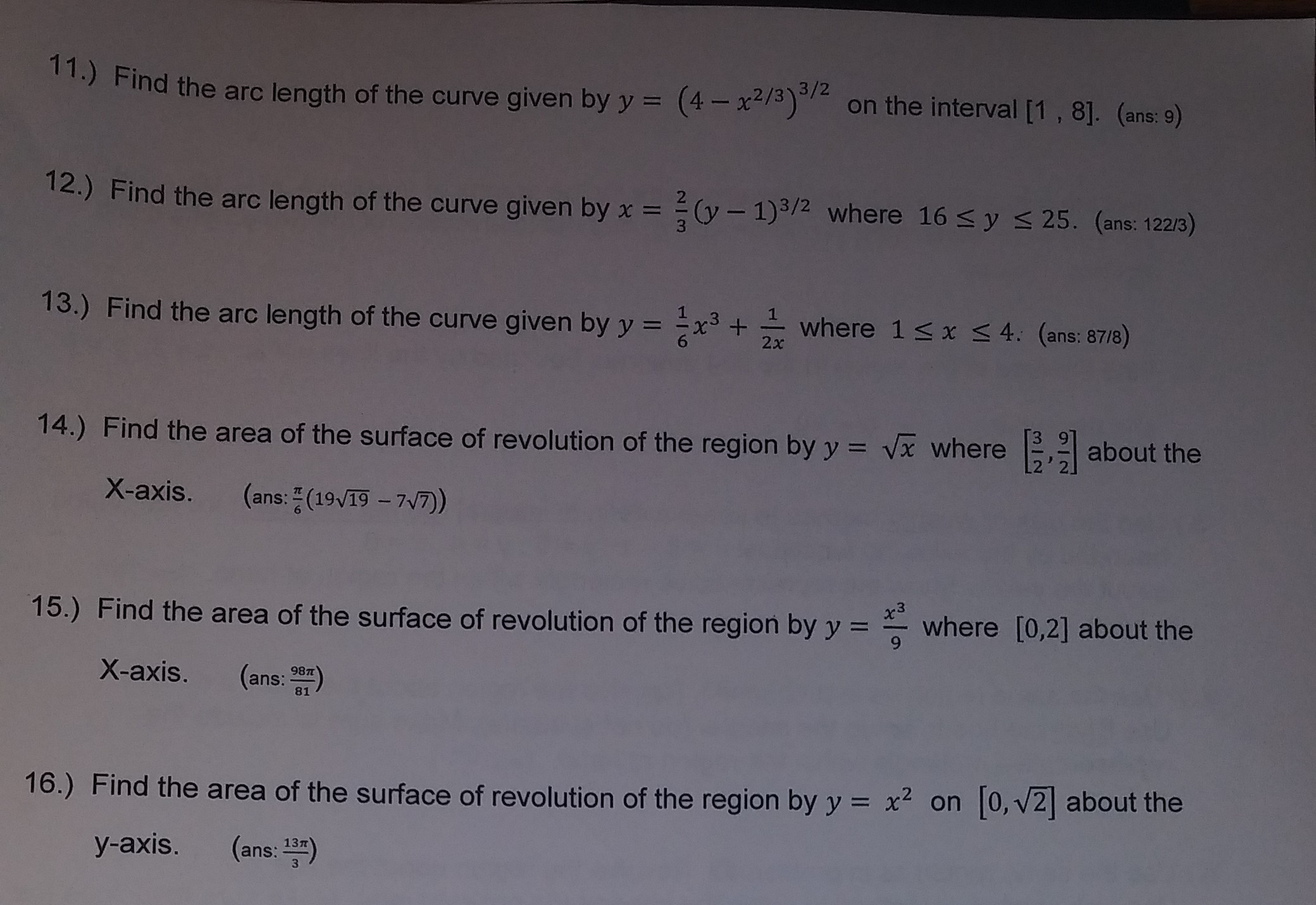# 11.) Find the arc length of the curve given by y (4on the interval [1, 81. (ome:-) 3/2 (4x2/3o on the interval [1, 8]. (ans: 9) Find the arc length of the curve given by x- 2 (y - 1)3/2 where 16 s y s 25. (ans: 122/3) 13.) Find the arc length of the curve given byyx where 1 s x 4. (ans: 8718) 14.) Find the area of the surface of revolution of the region by y vx where about the X-axis. (ans: (1917) 3 where [0,2] about the Find the area of the surface of revolution of the region by y X-axis. (ans: a) 15.) 16,) Find the area of the surface of revolution of the region by y 2 on [o,v2] about the y-axis. (ans: 13)

Question

Number 12help_outlineImage Transcriptionclose11.) Find the arc length of the curve given by y (4on the interval [1, 81. (ome:-) 3/2 (4x2/3o on the interval [1, 8]. (ans: 9) Find the arc length of the curve given by x- 2 (y - 1)3/2 where 16 s y s 25. (ans: 122/3) 13.) Find the arc length of the curve given byyx where 1 s x 4. (ans: 8718) 14.) Find the area of the surface of revolution of the region by y vx where about the X-axis. (ans: (1917) 3 where [0,2] about the Find the area of the surface of revolution of the region by y X-axis. (ans: a) 15.) 16,) Find the area of the surface of revolution of the region by y 2 on [o,v2] about the y-axis. (ans: 13) fullscreen

### Want to see this answer and more?

Experts are waiting 24/7 to provide step-by-step solutions in as fast as 30 minutes!*

*Response times vary by subject and question complexity. Median response time is 34 minutes and may be longer for new subjects.
Tagged in
MathCalculus

### Other